#### 期刊菜单

Research on Isolated Three-Phase AC Overvoltage Fault Detection Circuit Based on Bridge Uncontrolled Rectification
DOI: 10.12677/OJCS.2022.114004, PDF, HTML, XML, 下载: 54  浏览: 309  科研立项经费支持

Abstract: This paper presents an isolated three-phase AC overvoltage fault detection circuit based on bridge uncontrolled rectification. After analyzing the principle of the circuit, the Thevenin equivalent circuit model of the voltage divider rectifier circuit is established. According to the equivalent circuit model, the mathematical model of the three-phase AC voltage divider is derived, the first-order time-domain model is established, and the relevant parameters of the circuit are designed. Finally, a simulation model is built in PSIM and verified by simulation. The simulation results show that the circuit can transmit protection signals to DSP under abnormal operating voltage; no fault signal will be transmitted to DSP under normal operating voltage.

1. 引言

2. 拓扑结构及工作原理分析

2.1. 拓扑结构

2.2. 电路原理分析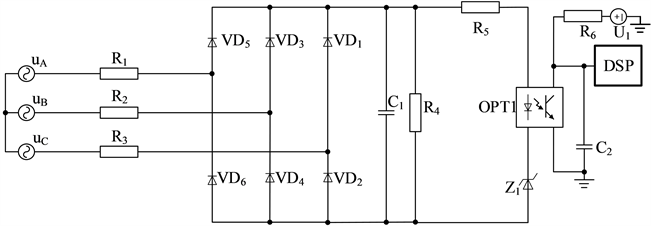Figure 1. Topology of isolated three-phase AC overvoltage fault detection circuit based on bridge uncontrolled rectification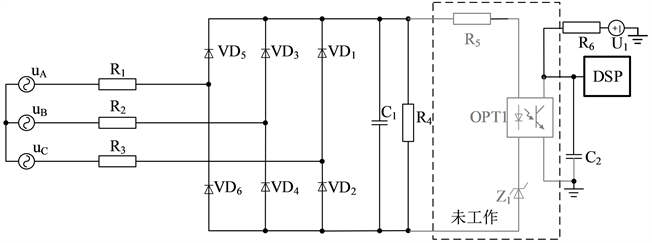Figure 2. Normal pressure working state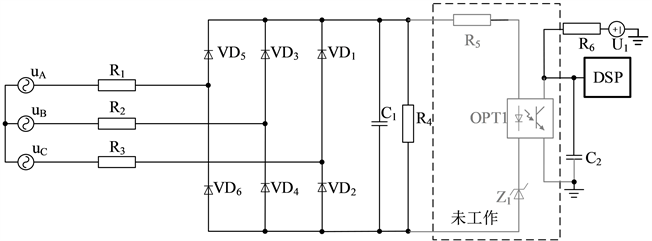Figure 3. Overvoltage working state

3. 分压桥式不控整流电路建模

3.1. 戴维南等效Figure 4. Phase A voltage is greater than phase B and phase C voltage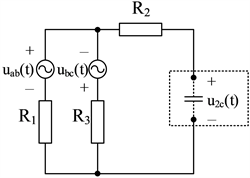Figure 5. Phase B voltage is greater than phase A and phase C voltage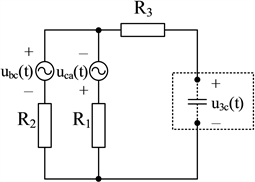Figure 6. Phase C voltage is greater than phase B and phase A voltage

$\left\{\begin{array}{l}{u}_{1c}\left(t\right)=|0.5\left({u}_{ca}\left(t\right)-{u}_{ab}\left(t\right)\right)|\\ {u}_{2c}\left(t\right)=|0.5\left({u}_{ab}\left(t\right)-{u}_{bc}\left(t\right)\right)|\\ {u}_{3c}\left(t\right)=|0.5\left({u}_{bc}\left(t\right)-{u}_{ca}\left(t\right)\right)|\end{array}$ (1)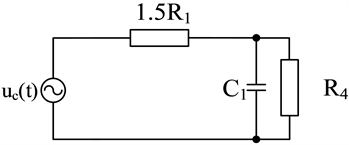Figure 7. Principle diagram of Thevenin’s equivalent circuit of voltage dividing circuit and bridge rectifier circuit

$\left\{\begin{array}{l}{u}_{dp1}\left(t\right)=\frac{{R}_{4}}{1.5{R}_{1}+{R}_{4}}{u}_{1c}\left(t\right)\\ {u}_{dp2}\left(t\right)=\frac{{R}_{4}}{1.5{R}_{1}+{R}_{4}}{u}_{2c}\left(t\right)\\ {u}_{dp3}\left(t\right)=\frac{{R}_{4}}{1.5{R}_{1}+{R}_{4}}{u}_{3c}\left(t\right)\end{array}$ (2)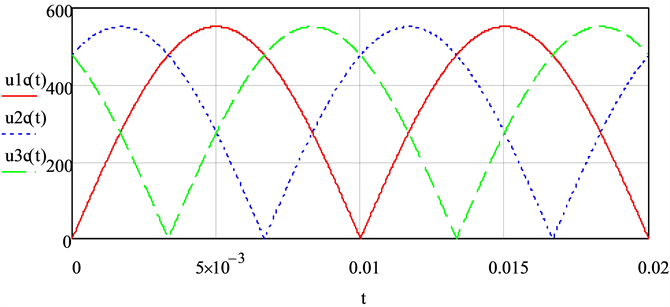Figure 8. Open circuit voltage in three states before voltage divisionFigure 9. Open circuit voltage in three states after voltage division

3.2. 时域分析

${V}_{z2}={V}_{z}+0.5$ (3)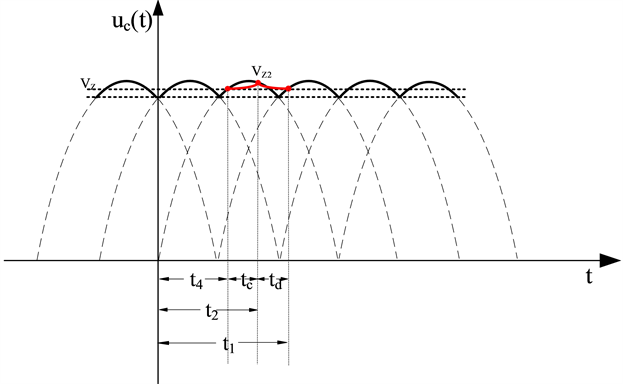Figure 10. Waveform diagram of charging voltage of bridge arm capacitor and actual voltage of bridge arm capacitor

${t}_{c}=\left(0.01-\frac{a\mathrm{sin}\left(\frac{{V}_{z2}}{{V}_{m}}\right)}{2\pi f}\right)-\frac{a\mathrm{sin}\left(\frac{{V}_{z}}{{V}_{m}}\right)}{2\pi f}$ (4)

${t}_{d}=\frac{a\mathrm{sin}\left(\frac{{V}_{z}}{{V}_{m}}\right)}{2\pi f}+\frac{0.01}{3}-\left(0.01-\frac{a\mathrm{sin}\left(\frac{{V}_{z2}}{{V}_{m}}\right)}{2\pi f}\right)$ (5)

$\left\{\begin{array}{l}{V}_{z2}={V}_{m}\left(1-{\text{e}}^{-\frac{{t}_{c}}{{\tau }_{1}}}\right)+{V}_{z}{\text{e}}^{-\frac{{t}_{c}}{{\tau }_{1}}}\\ {V}_{z}={V}_{z2}{\text{e}}^{-\frac{{t}_{d}}{{\tau }_{2}}}\\ {\tau }_{1}=\frac{1.5{R}_{1}{R}_{4}}{1.5{R}_{1}+{R}_{4}}{C}_{1}\\ {\tau }_{2}={R}_{2}{C}_{1}\end{array}$ (6)

4. 参数整定设计

${V}_{z}=16.5{\text{e}}^{-\frac{{t}_{d}}{{\tau }_{2}}}$ (7)

${V}_{z}=16.5{\text{e}}^{-\frac{{t}_{d}}{{\tau }_{2}}}$ (8)

5. 仿真验证Table 1. Performance index of fault detection circuitFigure 11. Simulation schematic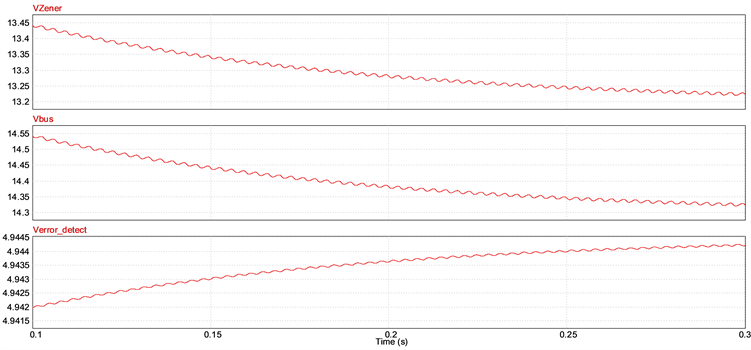Figure 12. Simulation oscillogramFigure 13. Simulation waveform under abnormal operating voltageTable 2. 220 V to 265 V simulation results

6. 结论

  Zhang, X., Wang, T.S. and Bao, B.C. (2021) A Detection Circuit for Improving the Unloading Transient Performance of the COT Controller. Electronics, 10, Article No. 2333. https://doi.org/10.3390/electronics10192333  黄小凡, 刘欣睿. 电压型不控整流电路[J]. 电焊机, 2017, 47(1): 56-62.  邓钧韬, 谭健, 吴特, 李权, 荣军. 电容滤波的三相不可控整流电路的仿真研究[J]. 船电技术, 2017, 37(12): 73-75+80.  沈瑶, 张舒媛, 冯媛媛, 邹建龙. 一阶RC电路实验教学改革与问题分析[J]. 实验室研究与探索, 2021, 40(12): 158-162.  Lu, S.Z., Deng, X.T. and Li, S.Q. (2020) A Simplified Zero-Voltage Switching Detection Circuit and Switching Loss Dynamic Optimisation Method for Synchronous Buck Converter. Electronics Letters, 57, 71-73. https://doi.org/10.1049/ell2.12065  Jalilian, A., Muttaqi, K.M. and Sutanto, D. (2021) A Novel Voltage Clamping-Based Overvoltage Protection Strategy to Avoid Spurious Trip of Inverter-Based Resources and Eliminate the Risk of Wildfire Following the REFCL Operation in Compensated Networks. IEEE Transactions on Industry Applica-tions, 57, 4558-4568. https://doi.org/10.1109/TIA.2021.3089116  Areerak, K., Sopapirm, T., Bozhko, S., Hill, C.I., Suyapan, A. and Areerak, K. (2018) Adaptive Stabilization of Uncontrolled Rectifier Based AC-DC Power Systems Feeding Constant Power Loads. IEEE Transactions on Power Electronics, 33, 8927-8935. https://doi.org/10.1109/TPEL.2017.2779541  Meng, F.G., Xu, X.N., Gao, L., Man, Z.C. and Cai, X.N. (2019) Dual Passive Harmonic Reduction at DC Link of the Double-Star Uncontrolled Rectifier. IEEE Transactions on Indus-trial Electronics, 66, 3303-3309. https://doi.org/10.1109/TIE.2018.2844840  Gable, R. (2021) An Overvoltage Protection Circuit. QST, 105, 36-37.